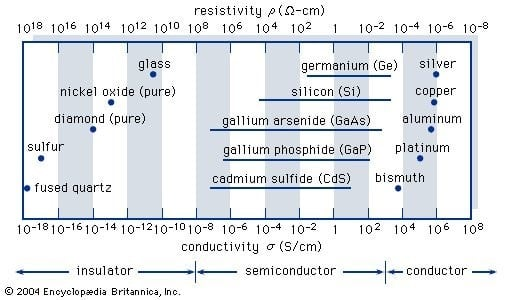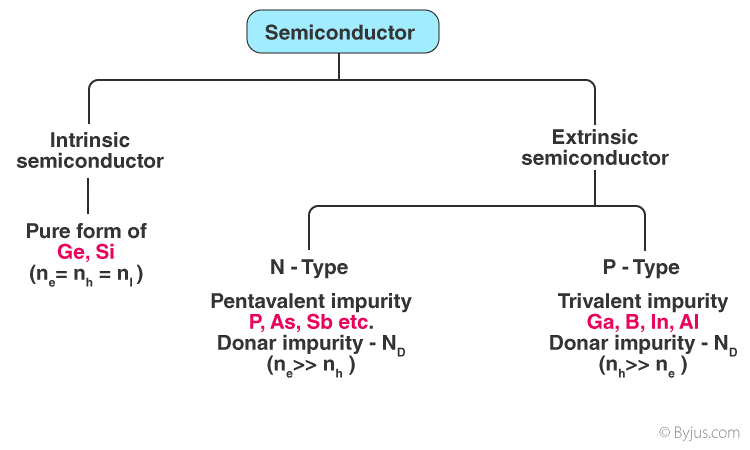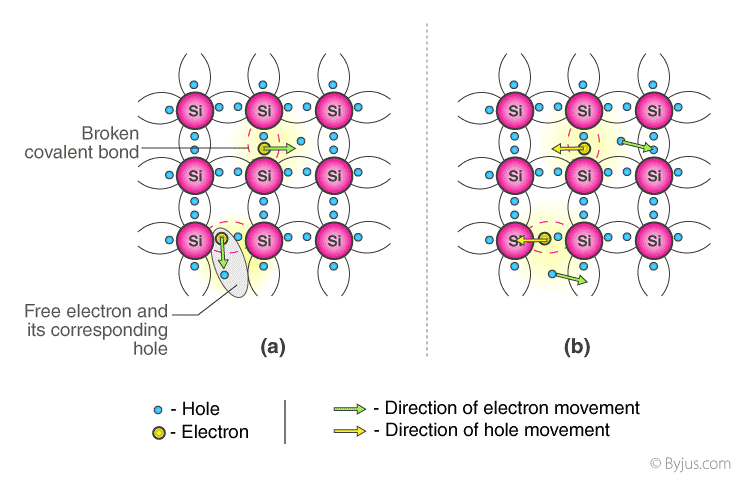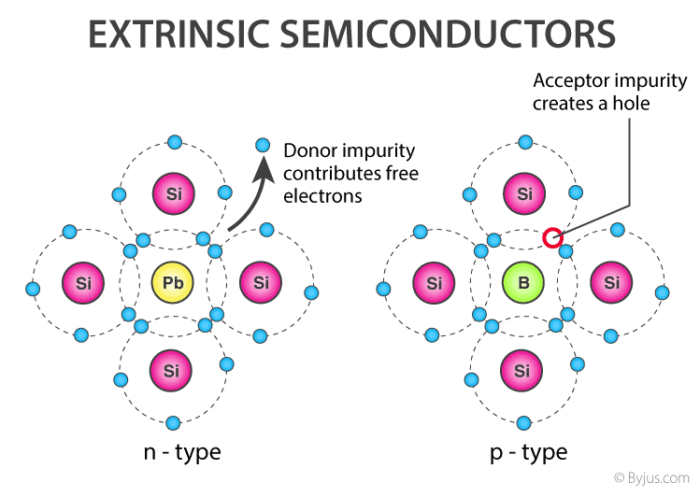# What are semiconductors? definition, types, industries.

The topic that we are going to discuss is 'semiconductor', a simple yet important and useful substance in the field of electronics.

A semiconductor is a material, typically a solid chemical element or compound, that can conduct electricity under certain conditions but not others, making it an excellent medium for controlling the flow of the electrical current. A semiconductor is a type of crystalline solid that is halfway between a conductor and an insulator in terms of electrical conductivity.

Insulators, semiconductors, and conductors are the three basic types of solid-state materials. (At low temperatures some conductors, semiconductors, and insulators can become superconductors.) The conductivities (and corresponding resistivities = 1/σ) associated with some essential materials in each of the three groups are shown in the diagram given below. Insulators, such as fused quartz and glass, have low conductivities of 10−18 to 10−10 siemens per centimeter, whereas conductors, such as aluminium, have high conductivities of 104 to 106 siemens per centimeter. Semiconductors' conductivities fall somewhere in between these two extremes and they're usually affected by temperature, light, magnetic fields and trace amounts of impurity atoms. The addition of about 10 atoms of boron (known as a dopant) per million atoms of silicon, for example, will increase its electrical conductivity by a thousandfold (partially accounting for the wide variability shown in the figure).#### Semiconductors' Characteristics:

Semiconductors have the ability to conduct electricity under optimal conditions. This distinguishes it as an excellent material for conducting electricity in a controlled manner. In contrast to conductors, charge carriers in semiconductors are generated solely by external energy (thermal agitation).

It allows a certain number of valence electrons to leap into the conduction band and cross the energy gap, leaving an equal number of unoccupied energy states, i.e. holes. The importance of electron and hole conduction is similar.

1. Resistivity: 10-5 to 106 Ω m.
2. Conductivity: 105 to 10-6 ohm/m.
3. Temperature resistance coefficient: Negative.
4. Electrons and holes trigger current flow.
5. Why does the resistance of semiconductors decrease as the temperature rises?

The difference in charge carrier density between conductors and semiconductors causes the difference in resistivity.

The resistivity of semiconductors decreases as the temperature rises because the number of charge carriers increases rapidly, resulting in a fractional shift in resistivity.

#### Some important properties:

Semiconductors have a variety of important properties, including:

1. At zero Kelvin, a semiconductor serves as an insulator. It functions as a conductor as the temperature increases.
2. Semiconductors can be doped to make the semiconductor devices ideal for energy conversion, switches, and amplifiers due to their exceptional electrical properties.
3. There are fewer power losses.
4. They have higher resistivity than conductors but a lower resistivity than insulators.
5. As the temperature increases, the resistance of semiconductor materials decreases, and vice versa.

#### Types of Semiconductors:

Semiconductors come in a number of shapes and sizes. Semiconductors are divided into two categories:

1. Intrinsic Semiconductor.
2. Extrinsic Semiconductor.#### 1. Intrinsic Semiconductor:

Chemically, an intrinsic form of semiconductor material is created to be very pure. There is only one form of a feature in it.(a) In absence of electric field. (b) In presence of electric Field.

The most common intrinsic semiconductor elements are germanium (Ge) and silicon (Si). They have four electrons in their valence shell (tetravalent). At absolute zero temperature, they form a covalent bond with the atom. Owing to collisions, a few electrons become unbounded and free to pass through the lattice as the temperature increases, resulting in an absence in its original location (hole).The conduction of electricity in the semiconductor is supported by these free electrons and holes. The number of negative and positive charge carriers is equal. Thermal energy will only ionise a few atoms in the lattice, resulting in lower conductivity.

Present flows in intrinsic semiconductors due to the motion of free electrons and holes. The total current is the sum of the thermally induced electron current Ie and the hole current Ih.

Ie + Ih = Complete Current (I)

#### 2. Extrinsic Semiconductor:

It is a type of semiconductor that has no intrinsic properties.

By adding a small number of suitable substitute atoms known as IMPURITIES, the conductivity of semiconductors can be greatly improved. DOPING is the method of introducing impurity atoms into a pure semiconductor. In a doped semiconductor, just 1 out of every 107 atoms is substituted by a dopant atom. Extrinsic semiconductors are further categorized into the following categories:

1. N-type Semiconductor.
2. P-type Semiconductor.#### N-type:

1. Mostly because of electrons.
2. Absolutely unchanged.
3. I = Ih and nh >> ne
4. Electrons are in the majority, and holes are in the minority.

When a pentavalent impurity (P, As, Sb, Bi) is doped into a pure semiconductor (Silicon or Germanium), four of the five valence electrons bond with the four electrons of Ge or Si.

The dopant's fifth electron is liberated. As a result, the impurity atom donates a free electron to the lattice for conduction and is called “Donar“.

#### P type:

1. Mainly because of the holes.
2. Entirely neutral.
3. I = Ih and nh >> ne.
4. Holes are in the majority, and electrons are in the minority.

When a pure semiconductor is doped with a trivalent impurity (B, Al, In, Ga), the impurity's three valence electrons bond with three of the semiconductor's four valence electrons.

S.No Intrinsic Semiconductor Extrinsic Semiconductor
1. Semiconductor in a pure form is called intrinsic semiconductor Semiconductor which are doped with impurity is called extrinsic semiconductor
2. Here the change carriers are produced only due to thermal agitation Here the change carriers are produced due to impurities and may also be produced due to thermal agitation
3. They have low electrical conductivity They have high electrical conductivity
4. They have low operating temperature They have high operating temperature
5. At 0K, Fermi level exactly lies betweeen condustion band and valence band At 0K, Fermi level exactly lies closer to conduction band in 'n' type semiconductor and lies near the valence band in 'p' type semiconductor
Examples: Si, Ge, etc. Examples: Si and Ge doped with Al, In, P, As etc.

#### Applications of semiconductors:

1. Semiconductors are used in a number of applications.
2. Let's look at how semiconductors are used in everyday life. Almost all electronic devices contain semiconductors. Our lives would be very different if they didn't exist.
3. Their dependability, compactness, low cost, and regulated conductivity are all advantages.

#### Semiconductors' Applications in Everyday Life:

1. Semiconductor devices are used to make temperature sensors.
2. They are used in 3D printers.
3. Microchips and self-driving vehicles use it.
4. Calculators, solar plates, computers, and other electronic devices use this material.
5. Semiconductors are used to produce transistors and MOSFET, which are used as switches in electrical circuits.

#### Semiconductors in Industrial Applications:

1. Semiconductors' physical and chemical properties allow them to create technological marvels such as microchips, transistors, LEDs, and solar cells.
2. Transistors and other controlling devices made of semiconductor materials create the microprocessor used to power the operation of space ships, trains, robots, and other devices.
HI, I am a student of Electrical Engineering. I am currently working for an electronic store. I work at the electrical components there. I am learning many useful practical concepts there. On the other hand, I give online tuitions to some students of high school I love electrical and electronics devices and have a plan to have a Master's Degree in Electronics.